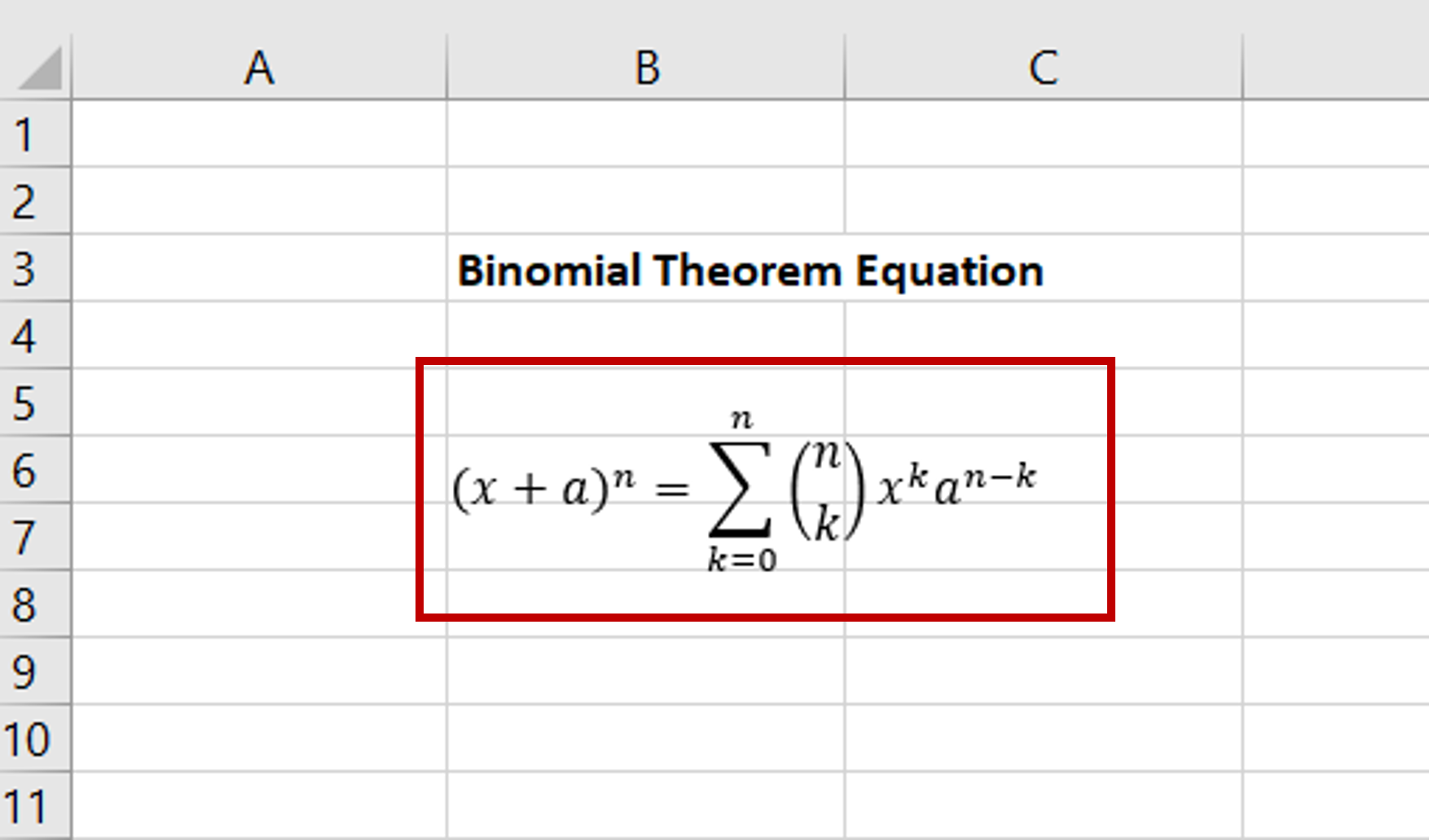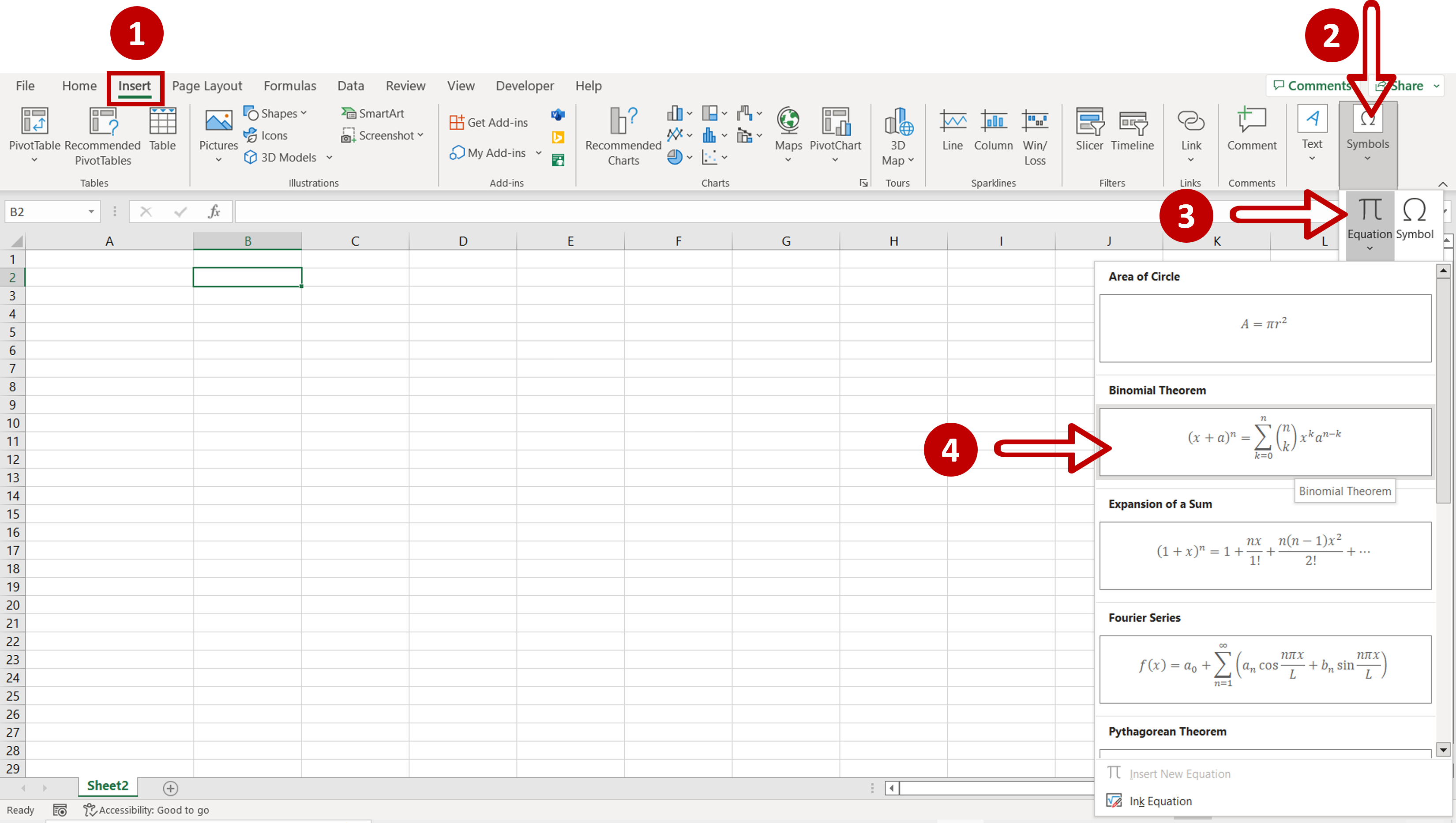# How to insert an equation in Excel

You can watch a video tutorial here.Excel is primarily used for its spreadsheet functions but it also supports several textual functions.  When evaluating an equation, for example, the area of a circle, you can do so by typing the formula in the cell using the equal sign (=). The problem arises when you want to include the equation itself. It becomes a complex process to include the Pi character or numerators and denominators within the cell. To solve this problem, Excel has some preset equations that can be used.

### Step 1 – Find the equation– Go to Insert > Symbols > Equation
– Select one of the preset equations or create your own using Ink Equation

### Step 2 – Move the equation to an appropriate location– The equation will be inserted into the file as an image
– Move the equation image to the desired location